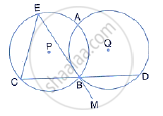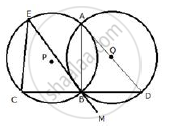Share

Circles with Centres P and Q Intersect at Points a and B as Shown in Figure. Cbd is a Segment and Ebm is Tangent to the Circle with Centre Q, at Point B. If the Circle Are Congruent; Show that Ce = Bd - ICSE Class 10 - Mathematics

ConceptTangent Properties - If a Line Touches a Circle and from the Point of Contact, a Chord is Drawn, the Angles Between the Tangent and the Chord Are Respectively Equal to the Angles in the Corresponding Alternate Segments

Question

Circles with centres P and Q intersect at points A and B as shown in the figure. CBD is a segment and EBM is tangent to the circle with centre Q, at point B. If the circle are congruent; show that
CE = BDSolutionJoin AB and AD
EBM is a tangent and BD is a chord.
∠DBM = ∠BAD (angles in alternate segments)
But, ∠DBM = ∠ CBE (Vertically opposite angles)
∴ ∠BAD = ∠CBE
Since in the same circle or congruent circles, if angles are equal, then chords opposite to them are also equal.
Therefore, CE = BD

Is there an error in this question or solution?

Video TutorialsVIEW ALL 

Solution Circles with Centres P and Q Intersect at Points a and B as Shown in Figure. Cbd is a Segment and Ebm is Tangent to the Circle with Centre Q, at Point B. If the Circle Are Congruent; Show that Ce = Bd Concept: Tangent Properties - If a Line Touches a Circle and from the Point of Contact, a Chord is Drawn, the Angles Between the Tangent and the Chord Are Respectively Equal to the Angles in the Corresponding Alternate Segments.
S